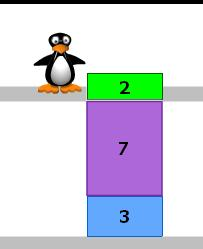Search IntMath
Close

# Yet another computer-based math system?

By Murray Bourne, 10 Jan 2008

The OCRegister's article Irvine man has kids loving math (the article has since disappeared, unfortunately) talks about the MIND Research Institute's ST Math Games program.

The background to this one is interesting. The founder of the program, Matthew Peterson, did not do well in school himself due to a problem with dyslexia.

His Flash based ST Math Games is largely 'language free' and aims at tweaking...

... spatial-temporal reasoning, the same reasoning used to learn music, to teach math concepts.

The process encourages students to visualise mathematical concepts (like adding fractions, solving equations) before they ever try to solve math problems using language, numbers or symbols.

The games revolve around the cartoon character Jiji, a penguin whose life is made easier when the student gets the problem correct.There are similarities between this program and the approach taken in Singapore to teach the solution of simultaneous equations using visualisation by blocks.

ST Math Games is an interesting approach that is worth investigating. Apart from anything else, it does not insist that students start abstracting before they have a concrete understanding of concepts and it is catering for those who struggle with mathematics because of language issues.

### Comment Preview

HTML: You can use simple tags like <b>, <a href="...">, etc.

To enter math, you can can either:

1. Use simple calculator-like input in the following format (surround your math in backticks, or qq on tablet or phone):
a^2 = sqrt(b^2 + c^2)
(See more on ASCIIMath syntax); or
2. Use simple LaTeX in the following format. Surround your math with $$ and $$.
$$\int g dx = \sqrt{\frac{a}{b}}$$
(This is standard simple LaTeX.)

NOTE: You can mix both types of math entry in your comment.